Test: Digital Communications - 2

# Test: Digital Communications - 2 - Electronics and Communication Engineering (ECE)

Test Description

## 20 Questions MCQ Test GATE ECE (Electronics) 2023 Mock Test Series - Test: Digital Communications - 2

Test: Digital Communications - 2 for Electronics and Communication Engineering (ECE) 2023 is part of GATE ECE (Electronics) 2023 Mock Test Series preparation. The Test: Digital Communications - 2 questions and answers have been prepared according to the Electronics and Communication Engineering (ECE) exam syllabus.The Test: Digital Communications - 2 MCQs are made for Electronics and Communication Engineering (ECE) 2023 Exam. Find important definitions, questions, notes, meanings, examples, exercises, MCQs and online tests for Test: Digital Communications - 2 below.
Solutions of Test: Digital Communications - 2 questions in English are available as part of our GATE ECE (Electronics) 2023 Mock Test Series for Electronics and Communication Engineering (ECE) & Test: Digital Communications - 2 solutions in Hindi for GATE ECE (Electronics) 2023 Mock Test Series course. Download more important topics, notes, lectures and mock test series for Electronics and Communication Engineering (ECE) Exam by signing up for free. Attempt Test: Digital Communications - 2 | 20 questions in 60 minutes | Mock test for Electronics and Communication Engineering (ECE) preparation | Free important questions MCQ to study GATE ECE (Electronics) 2023 Mock Test Series for Electronics and Communication Engineering (ECE) Exam | Download free PDF with solutions
 1 Crore+ students have signed up on EduRev. Have you?
Test: Digital Communications - 2 - Question 1

### The Autocorrelation function is given by exp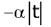. Then the PSD is given by

Test: Digital Communications - 2 - Question 2

### X is a binary memory less source with p (x = 0) = 0.3. If the source is transmitted over a BSC with cross-over probability = 0.1, then the capacity of the channel is

Detailed Solution for Test: Digital Communications - 2 - Question 2

Capacity of the channel is

C = 1 -H(0.1)=1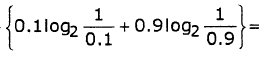= 1 -0.469 = 0.531

Test: Digital Communications - 2 - Question 3

### Two binary random variables X and Y are distributed according to the joint distributions p(x = y = 0) = p(x = 0, y = 1) = p(x = y = 1) = 1/3 . Then the entropy H(x) is

Detailed Solution for Test: Digital Communications - 2 - Question 3

The marginal probabilities are given by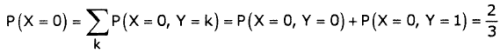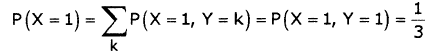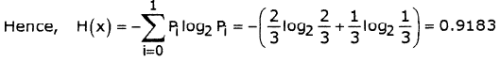*Answer can only contain numeric values
Test: Digital Communications - 2 - Question 4

In a Binary digital communication system, the threshold voltage of comparator is 'x' volts and voltage at input of comparator is uniformly distributed in the range of x-0.5 to x+0.25 when '0' is transmitted and it is in the range x-0.25 to x+0.5 when bit '1' is transmitted, the average probability of error in the system is_____________

Detailed Solution for Test: Digital Communications - 2 - Question 4

Probability density function of f (z/0) is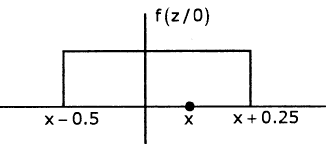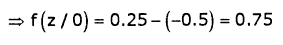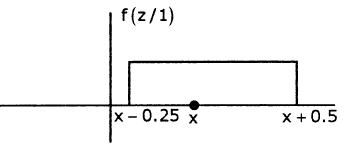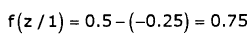When '0' is transmitted, it will be detected as '1' with probability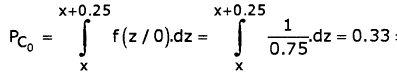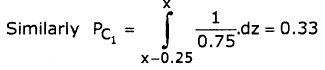Average probability of error = 0.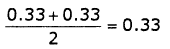Test: Digital Communications - 2 - Question 5

If probability of error of PSK signal is 'x', then what will be probability of error of FSK and ASK signal. (Assume x =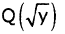Detailed Solution for Test: Digital Communications - 2 - Question 5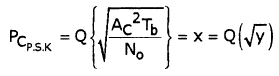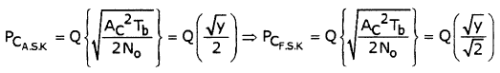As Q-function is not linear operator, we cannot find the probability errors of FSK and ASK with probability error of PSK

Test: Digital Communications - 2 - Question 6

If input to matched filter is s(t) = f(t)+ 2f (t)δ(t - 0.5) + f(t - 2.5)*8δ(t+ 0.5)

where f(t) is shown below, then determine the impulse response of matched filter h(t) ; 2 5 ≤ t < 3

(Note: δ is Delta function and * is convolution operator)

Detailed Solution for Test: Digital Communications - 2 - Question 6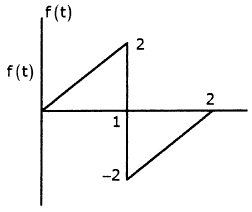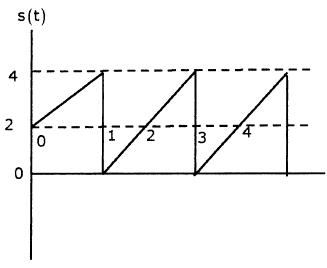2f (t)6(t - 0.5) = 2f(0.5) = 2x1 = 2 f(t-2.5)*6(t+ 0.5) = f(t-(2.5- 0.5))

=f(t-2)

s(t)=f(t)+f(t-2)+2

= -2f +6 ; 2 5 t 5 3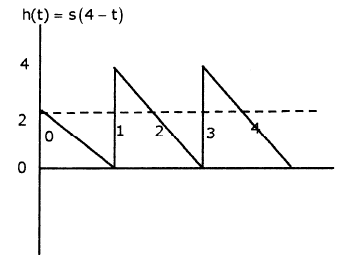Test: Digital Communications - 2 - Question 7

Match the following:

Modulation             Type of signaling format to be

technique                     used for modulation

4. DPSK

5. QPSK

Detailed Solution for Test: Digital Communications - 2 - Question 7

A.S.K signal will be present when 11' is transmitted and absent when '0' is transmitted hence ON -

OFF

In P.S.K for phase reversal a non return to zero format will be used

In FSK

fi (t) = fc (t)+ kfAv             for '1'

fc (t) kfAv              for '0'

In D.P.S.K the output of differential enconder will be in ON - OFF format which is than modified using Amplitude level shifting to get NRZ format

In Q.P.S.K

For '10'

Ac cos 27tfct - Ac si n 2/rfct . Hence NRZ

*Answer can only contain numeric values
Test: Digital Communications - 2 - Question 8

Consider a BSC with cross-over probability of error P. Suppose that R is the number of bits in a source code word that represents one of 2R possible levels at the output of quantizer. If R = 5, P = 0.01, then the probability of having 5 or less bits in error in a code word is_____________

Detailed Solution for Test: Digital Communications - 2 - Question 8

P(5 or less errors in 5 bits) =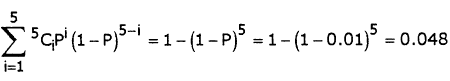*Answer can only contain numeric values
Test: Digital Communications - 2 - Question 9

A black and white television picture may be viewed as consisting of approximately 3x105 elements, each of which may occur one of 10 distinct brightness levels with equal probability. Transmission rate is 30 picture frames/ second and SNR is 30 dB. Then the minimum bandwidth required to support the transmission of the resulting video signal is   MHz

Detailed Solution for Test: Digital Communications - 2 - Question 9

The information in each element is log210 bits. The information in each picture is [31og210]x105 bits. The transmitted information rate is

[9 log210] x106bits / second.

The channel must have this capacity. From the information capacity theorem,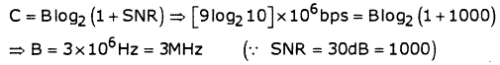Test: Digital Communications - 2 - Question 10

A random variable X has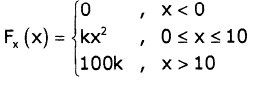Q. The value of constant K is

Detailed Solution for Test: Digital Communications - 2 - Question 10

fx(x) = 2kx ; 0 ≤ x ≤ 10  ⇒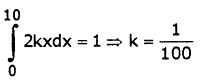Test: Digital Communications - 2 - Question 11

A random variable X hasQ. The value of P(4 < x 5 ≤ 8) is

Detailed Solution for Test: Digital Communications - 2 - Question 11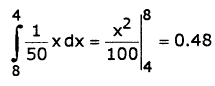Test: Digital Communications - 2 - Question 12

A telegraphic source has two symbols dot and dash. The dot duration is 0.2sec. The dash is twice as long as the dot and half as probable.

Q. The entropy of source is

Detailed Solution for Test: Digital Communications - 2 - Question 12

P(Dot) + P(Dash) =1

P(Dot)+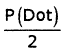= 1

P(Dot) = 2/3P(Dash) = 1/3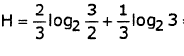= 0.92 bits / symbol

Test: Digital Communications - 2 - Question 13

A telegraphic source has two symbols dot and dash. The dot duration is 0.2sec. The dash is twice as long as the dot and half as probable.

Q. The information rate is

Detailed Solution for Test: Digital Communications - 2 - Question 13

Number of symbols / sec =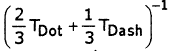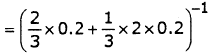= 3.75

Information rate = 3.75 x0.92= 3.45bits / sec

Test: Digital Communications - 2 - Question 14

Consider a cascaded block of an LTI system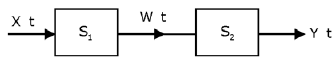The impulse response of S1. is h1. t = δ t -2 The impulse response of 52 is h2 t = t.e-ru t

The input x(t) is wide sense stationary random process with Rxx 0 = 3 and variance = 1

Q. The mean of the random process w(t) is

Detailed Solution for Test: Digital Communications - 2 - Question 14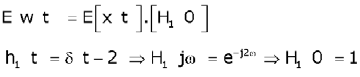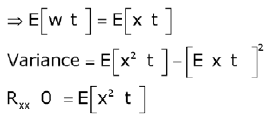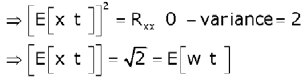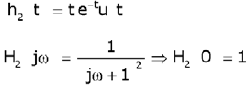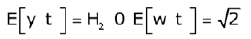Test: Digital Communications - 2 - Question 15

Consider a cascaded block of an LTI systemThe impulse response of S1. is h1. t = δ t -2 The impulse response of 52 is h2 t = t.e-ru t

The input x(t) is wide sense stationary random process with Rxx 0 = 3 and variance = 1

Q. If w(t) is input to system 52, then mean of process Y(t) will be

Detailed Solution for Test: Digital Communications - 2 - Question 15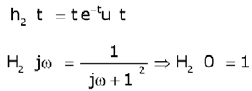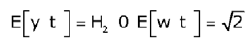Test: Digital Communications - 2 - Question 16

CDF of a random variable x is given below.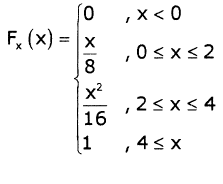Q. E[x] is

Detailed Solution for Test: Digital Communications - 2 - Question 16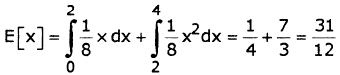Find PDF from CDF, PDF =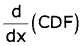Test: Digital Communications - 2 - Question 17

CDF of a random variable x is given below.Q. Variance is

Detailed Solution for Test: Digital Communications - 2 - Question 17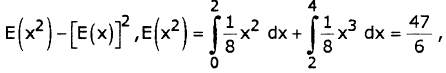Variance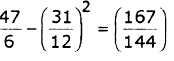Test: Digital Communications - 2 - Question 18

Consider a discrete memory less source X with two symbols x1 and x2 and P (xi) = 0.9, P (x2) = 0.1. These symbols are encoded as below.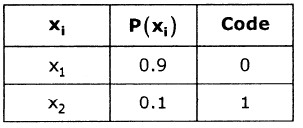Q. Find the source efficiency η

Detailed Solution for Test: Digital Communications - 2 - Question 18

average code length L per symbol is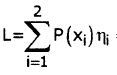= (0.9)1 + (0.1)1 = 1bit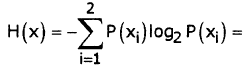-0.9 log2 0.9 - 0.1log2 0.1 = 0.469bits / symbol

code efficiency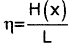= 0.469 = 46.9%

Test: Digital Communications - 2 - Question 19

Consider a discrete memory less source X with two symbols x1 and x2 and P (xi) = 0.9, P (x2) = 0.1. These symbols are encoded as below.Q. Find the redundancy γ

Detailed Solution for Test: Digital Communications - 2 - Question 19

redundancy r = 1- η =1- 0.469 = 0.531 = 53.1%

Test: Digital Communications - 2 - Question 20

The input to a linear delta modulator having a step-size D = 0.628 is a sine wave with frequency fm and peak amplitude Em. If the sampling frequency fs = 40kHz, the combination of the sine-wave frequency and peak amp, where slope overload will take place is

Em                   fin                                         Em                    fin

(a)           0.3V               8kHz                            (b)       1.5V                 4kHz

(c)          1.5V               2kHz                            (d)       3.0V                 1kHz

## GATE ECE (Electronics) 2023 Mock Test Series

21 docs|263 tests
Information about Test: Digital Communications - 2 Page
In this test you can find the Exam questions for Test: Digital Communications - 2 solved & explained in the simplest way possible. Besides giving Questions and answers for Test: Digital Communications - 2, EduRev gives you an ample number of Online tests for practice

## GATE ECE (Electronics) 2023 Mock Test Series

21 docs|263 tests(Scan QR code)